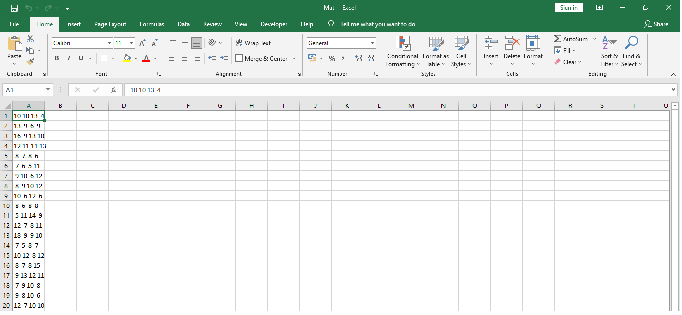# How to save a matrix as CSV file using R?

To save a matrix as CSV file using R, we can use write.matrix function of MASS package. For Example, if we have a matrix called M and we want to save it as CSV file then we can use the below mentioned command −

write.matrix(M,file="Mat.csv")

## Example

Following snippet creates a sample matrix −

M<-matrix(rpois(80,10),ncol=4)
M

The following matrix is created −

   [,1] [,2] [,3] [,4]
[1,] 10 10   13   4
[2,] 13  9    6   9
[3,] 16  9   13  10
[4,] 12 11   11  13
[5,] 8   7    8   6
[6,] 7   6    5  11
[7,] 9  10    6  12
[8,] 8   9   10  12
[9,] 10  6   12   6
[10,] 8   6    8   8
[11,] 5  11   14   9
[12,] 12  7    8  11
[13,] 18  9    9  10
[14,] 7   5    8   7
[15,] 10 12    8  12
[16,] 8   7    8  15
[17,] 9  13   12  11
[18,] 7   9   10   8
[19,] 9   8   10   6
[20,] 12  7   10  10

To load MASS package and save matrix M as CSV file on the above created matrix, add the following code to the above snippet −

M<-matrix(rpois(80,10),ncol=4)
library(MASS)
write.matrix(M,file="Mat.csv")


## Output

If you execute all the above given snippets as a single program, it generates the following Output −